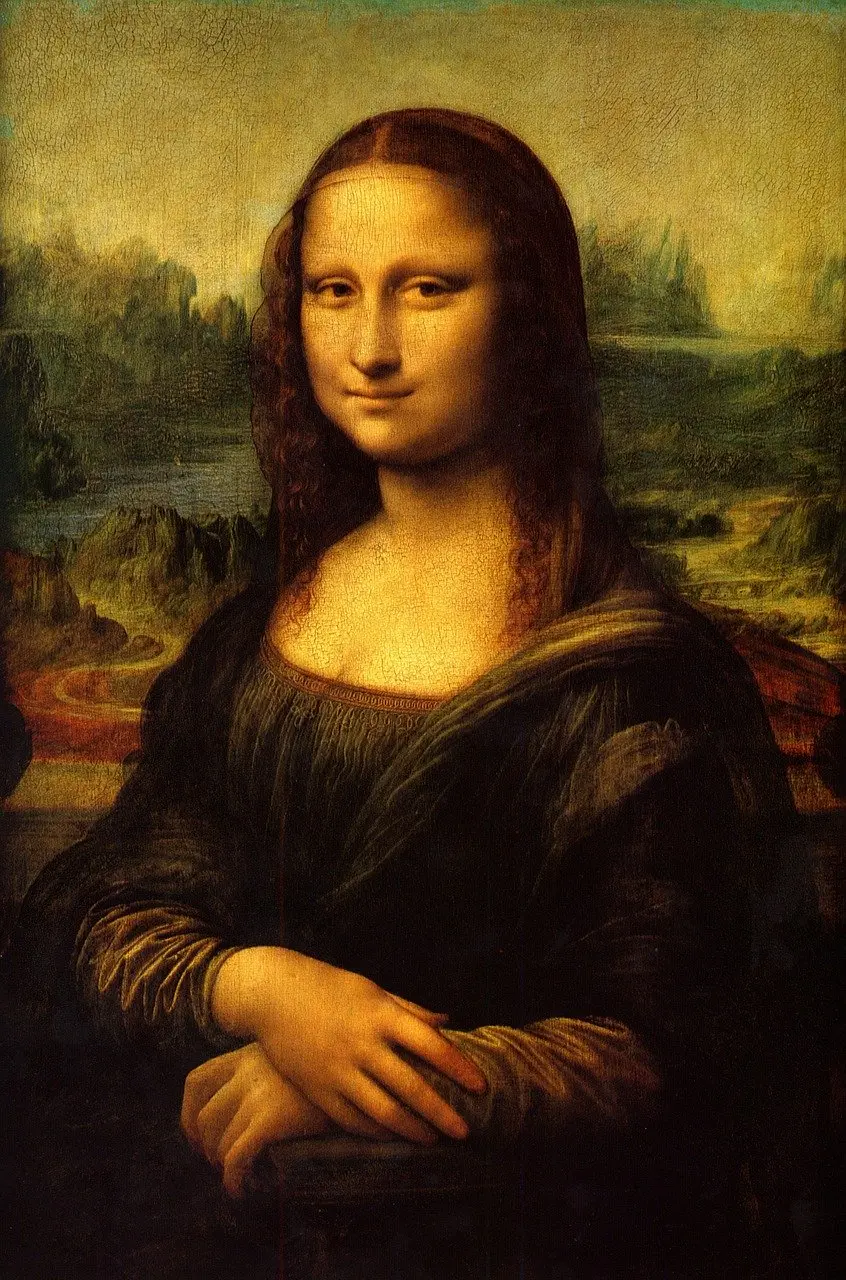# 用Python在Excel里画出蒙娜丽莎

## 前言

PS：如有需要Python学习资料的小伙伴可以加点击下方链接自行获取## PIL使用

PIL是Python里面做图像处理的时候十分常用的一个库，功能也是十分的强大，这里只需要用到PIL里一小部分的功能。

```1 from PIL import Image
2 img = Image.open(img_path) # 读取图片
3 width, height = img.size # 获取图片大小
4 r, g, b = img.getpixel((w - 1, h - 1)) # 获取像素色彩值```

• `Image.open()`是PIL里面打开一张图片的函数，支持多种图片类型

• `img_path`是图片路径，可以是相对路径，也可以是绝对路径

• `img.size`是获取图片的size属性，包含图片的宽和高

• `img.getpixel()`是获取图片色彩值的函数，需传入一个tuple或list，值为像素坐标xy

## openpyxl使用

`openpyxl`几乎是Python里功能最全的操作excel文件的库了，这里也只需要用到它的一小部分功能。

```1 import openpyxl
2 from openpyxl.styles import fills
3 ​
4 workbook = openpyxl.Workbook()
5 worksheet = workbook.active
6 cell.fill = fills.PatternFill(fill_type="solid", fgColor=hex_rgb)
7 workbook.save(out_file)```

• `openpyxl.Workbook()`新建一个excel文件

• `workbook.active` 激活一个工作表

• `cell.fill = fills.PatternFill(fill_type="solid", fgColor=hex_rgb)`填充一个单元格，`fill_type="solid"`是填充类型，`fgColor=hex_rgb`是填充的颜色

• `workbook.save()`保存文件，需传入要保存的文件名

## 写一段代码

`写这一个画图的需求需要用到的核心就是上面介绍的PIL跟openpyxl的几种用法。但是在实际写的时候，还会有一些其他问题，比如：`

1、getpixel()获取的颜色值是rgb十进制的，但fills.PatternFill 里的fgColor`参数接收到的颜色值是十六进制的值 这个问题其实就是十进制转十六进制，很容易解决

```1 def int_to_16(num):
2     num1 = hex(num).replace('0x', '')
3     num2 = num1 if len(num1) > 1 else '0' + num1 # 位数只有一位的时候在前面补零
4     return num2```

2、excel的单元格默认是长方形，修改为正方形才不会使图片变形

```1 if h == 1:
2   _w = cell.column
3   _h = cell.col_idx
4   # 调整列宽
5   worksheet.column_dimensions[_w].width = 1
6 ​
7 # 调整行高
8 worksheet.row_dimensions[h].height = 6```

3、excel支持的样式数量有限``` 1 MAX_WIDTH = 300
2 MAX_HEIGHT = 300
3 def resize(img):
4     w, h = img.size
5     if w > MAX_WIDTH:
6         h = MAX_WIDTH / w * h
7         w = MAX_WIDTH
8 ​
9     if h > MAX_HEIGHT:
10         w = MAX_HEIGHT / h * w
11         h = MAX_HEIGHT
12     return img.resize((int(w), int(h)), Image.ANTIALIAS)```

## 全部代码

``` 1 # draw_excel.py
2 ​
3 from PIL import Image
4 import openpyxl
5 from openpyxl.styles import fills
6 import os
7 ​
8 MAX_WIDTH = 300
9 MAX_HEIGHT = 300
10 ​
11 def resize(img):
12     w, h = img.size
13     if w > MAX_WIDTH:
14         h = MAX_WIDTH / w * h
15         w = MAX_WIDTH
16 ​
17     if h > MAX_HEIGHT:
18         w = MAX_HEIGHT / h * w
19         h = MAX_HEIGHT
20     return img.resize((int(w), int(h)), Image.ANTIALIAS)
21 ​
22 ​
23 def int_to_16(num):
24     num1 = hex(num).replace('0x', '')
25     num2 = num1 if len(num1) > 1 else '0' + num1
26     return num2
27 ​
28 ​
29 def draw_jpg(img_path):
30 ​
31     img_pic = resize(Image.open(img_path))
32     img_name = os.path.basename(img_path)
33     out_file = './result/' + img_name.split('.') + '.xlsx'
34     if os.path.exists(out_file):
35         os.remove(out_file)
36 ​
37     workbook = openpyxl.Workbook()
38     worksheet = workbook.active
39 ​
40     width, height = img_pic.size
41 ​
42     for w in range(1, width + 1):
43 ​
44         for h in range(1, height + 1):
45             if img_pic.mode == 'RGB':
46                 r, g, b = img_pic.getpixel((w - 1, h - 1))
47             elif img_pic.mode == 'RGBA':
48                 r, g, b, a = img_pic.getpixel((w - 1, h - 1))
49 ​
50             hex_rgb = int_to_16(r) + int_to_16(g) + int_to_16(b)
51 ​
52             cell = worksheet.cell(column=w, row=h)
53 ​
54             if h == 1:
55                 _w = cell.column
56                 _h = cell.col_idx
57                 # 调整列宽
58                 worksheet.column_dimensions[_w].width = 1
59             # 调整行高
60             worksheet.row_dimensions[h].height = 6
61
62             cell.fill = fills.PatternFill(fill_type="solid", fgColor=hex_rgb)
63 ​
64         print('write in:', w, '  |  all:', width + 1)
65     print('saving...')
66     workbook.save(out_file)
67     print('success!')
68 ​
69 if __name__ == '__main__':
70     draw_jpg('mona-lisa.jpg')```

https://www.cnblogs.com/Qqun821460695/p/11941700.html

「点点赞赏，手留余香」

还没有人赞赏，快来当第一个赞赏的人吧！

TensorFlow
0 条回复 A 作者 M 管理员
所有的伟大，都源于一个勇敢的开始！